# Tutorial: Create calculated fields in Data Studio

Learn by doing: perform arithmetic and use conditional expressions.

## Goals

• Learn how to create a simple arithmetic calculated field.
• Create a conditional expression to evaluate your data and return different results.
• Learn different approaches to implementing calculated fields.

## Scenario

Suppose you manage an online pet supply store. You might measure sales data, such product codes (SKU), item names, price, and quantity sold. Here's a simple example:

 SKU Item Price Qty Sold D-001 Healthy Dog Dog Food 79.96 16 B-002 Parrot Perch 74.97 8 B-001 Pretty Bird Bird Seed 31.96 20 C-002 Hungry Kitty Cat Food 29.98 3 D-002 Playful Puppy Toy 17.97 11 C-001 Happy Cat Catnip 14.97 4

Calculated fields in Data Studio can help you manipulate and extend this data. In this exercise, you'll use calculated fields to:

• calculate the total value of each order
• apply a discount to orders over a certain amount
• categorize sales into departments.

### A note on the solutions

There can often be more than one way to achieve a particular result with calculated fields, depending on your needs and how you want to use those fields. This tutorial walks you through one approach (using separate fields for the calculations). At the end of this article, you'll find some alternative solutions.

## Sample data

To complete this exercise as written, use the sample data below. Otherwise, use your own data (adjusting the field names accordingly). You'll need access to Google Sheets. Alternatively, you could use the File Upload connector to import the data into Data Studio.

Copy and paste this data into a text file:

```SKU,Item,Price,Qty Sold D-001,Healthy Dog Dog Food,79.96,16 B-002,Parrot Perch,74.97,8 B-001,Pretty Bird Bird Seed,31.96,20 C-002,Hungry Kitty Cat Food,29.98,3 D-002,Playful Puppy Toy,17.97,11 C-001,Happy Cat Catnip,14.97,4```

##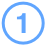Prepare the data

Goal: Create a Google Sheet using the sample data above.
1. Copy the sample data in the box above.
2. Open a text editor, paste in the sample data, then save the file as `pet-store-data.csv`.
• Be sure to save this file as plain text, otherwise Sheets won't be able to import the data.

• On Windows, you can use Notepad.

• On Mac OS, you can use TextEdit, then select Format > Make plain text.

• On Chrome OS, you can use the Text app, or Docs and then select File > Download and choose Plain Text.

• Create a new Google Sheet.
• In Sheets, select File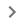Import.
• Select Upload, then upload the sample data file you created in step 2.

##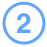Create a report and add the data

Goal: Connect a data source to the Pet Store spreadsheet and add it to a report.
1. Create a new report in Data Studio.
2. In the Add data to report panel, select the Google Sheets connector.
3. Select the `Pet Store` spreadsheet and worksheet you just created.
4. Leave the default options selected.
5. In the lower right, click ADD.

In a moment, you'll see the new report, with a table on the canvas that uses fields from the Pet Store data source.

##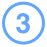Create the subtotal field

Goal: Create a field that calculates the order subtotal.
You can create 2 kinds of calculated fields: chart-specific calculated fields and data source calculated fields. In this tutorial, you'll create data source calculated fields. The main benefits of data source calculated fields are that you can use them in any chart based on this data source, and that they can refer to other calculated fields.

2. To the right of the Pet Store data source, click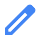EDIT.
The data source fields panel appears.
3. On the top right, click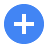ADD A FIELD.
The calculated field editor appears.
4. In Field Name, enter `Subtotal`.
5. In Formula, enter `Price * Qty Sold`.
Tip: The formula editor autocompletes dimension, metric, or function names as you type. You can also drag fields from the Available Fields list on the left into your formula.
6. On the bottom right, click SAVE.
7. On the left, return to the main data source editor by clicking ALL FIELDS.

##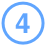Create the discount field

Goal: Create a field that applies a variable discount rate to orders of a certain value.

Let's say you want to give different discount rates depending on the value of the order. Create another calculated field, called `Discount`, that evaluates the Subtotal field and applies the following discounts.

 Subtotal value Discount rate 0 - 99 0 100 - 499 5% 500 or more 10%

Hints:

• In this step, you'll only calculate the discount. You'll apply it in the following step by multiplying the Subtotal field by the Discount field.
• Use the CASE expression to evaluate the Subtotal field.
• Use a WHEN clause for each discount rate.
• Use an ELSE clause to return the full price (0% discount).
• Express the discount as a decimal value. For example, a 25% discount would be .25.

## Solution

```CASE     WHEN Subtotal > 499 THEN .9     WHEN Subtotal > 100 THEN .95     ELSE 1 END```

Note: WHEN clauses are evaluated in order, and the first one that is true is the one whose THEN clause is returned.

##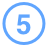Create the total field

Goal: Create a field that applies the discount rate to the subtotal.

Create another calculated field, called `Total`, that multiples the Subtotal field by the Discount field.

## Solution

`Subtotal * Discount`

##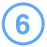Create the department field

Goal: Create a calculated field that categorizes items sold into departments.

Create a final calculated field, called Department, that parses the SKU field and assigns new values depending on the SKU. You'd like to group dog, cat, and bird related items into separate departments.

Hint: use the `CASE` statement again, this time, with the STARTS_WITH (or REGEXP_MATCH) function.

## Solution

```CASE     WHEN STARTS_WITH(SKU, "C") THEN "Cat"     WHEN STARTS_WITH(SKU, "D") THEN "Dog"     WHEN STARTS_WITH(SKU, "B") THEN "Bird"     ELSE "Misc" END```

##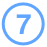See your data in a report

1. After saving the Department field, on the left, return to the data source editor by clicking ALL FIELDS.
2. In the upper right, click DONE.
3. On the right close the manage data sources panel.

1. Select the table.
2. On the right, drag each calculated field you created from the Available Fields list to the dimension and metrics section of the Data panel, as shown below: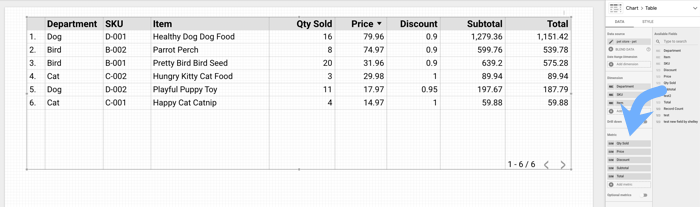## Alternative solutions

The solution above breaks the problem up into individual chunks and create discrete fields to handle each chunk, as you've done with the `Subtotal`, `Discount`, and `Total` fields.

Another approach is to perform all of the calculations in a single calculated field. For example, you could combine steps 3 - 5 of this tutorial into one field:

```CASE     WHEN Price * Qty Sold > 499 THEN (Price * Qty Sold) * .9     WHEN Price * Qty Sold > 100 THEN (Price * Qty Sold) * .95     ELSE Price * Qty Sold END```

There are pros and cons to each approach:

Breaking down complex formulas into separate fields can make your formulas easier to read and write, and less error-prone. Separate fields can also be useful in more contexts. For example, using a separate field for the discount rate lets you use that field in other calculations or display it in your reports. One drawback to creating formulas with lots of discrete calculated fields is that it may not be obvious what each one does, so you'll need to edit them individually to see how they are calculated or make changes.

On the other hand, centralizing all the logic into one field can make simple formulas easier to understand and edit. But you also might have to do a lot of repetitive typing, and a complex formula may be harder to extend or maintain. For example, say you had 10 product tiers with different order quantity thresholds? Or say you wanted to use the same discounts for a different product line? In these cases, it might be easier or more efficient to use separate fields for those thresholds and discounts.

### The middle path

This solution blends the 2 approaches described above. It keeps the main logic in a single field, while breaking out some of the dependent logic into separate fields:

```CASE     WHEN Subtotal > Large Order THEN Subtotal * Large Order Discount     WHEN Subtotal > Medium Order THEN Subtotal * Medium Order Discount     ELSE Subtotal END```

For this solution, we've created separate fields to hold values for large and medium sized orders and corresponding discounts.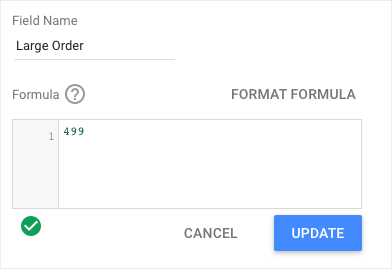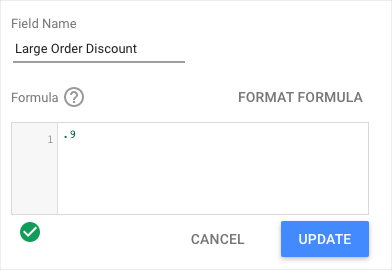This solution has the advantage of being easy to read, flexible, and relatively easy to adjust as needed.